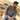# Validate email address using regex in JavaScriptTaimoor Sattar

We can define regular expressions to match the patterns in strings. To validate the email address, we can define the regex expression for email validation as below.

``````function validateEmail (email) {
const re = /^(([^<>()\[\]\\.,;:\s@"]+(\.[^<>()\[\]\\.,;:\s@"]+)*)|(".+"))@((\[[0-9]{1,3}\.[0-9]{1,3}\.[0-9]{1,3}\.[0-9]{1,3}\])|(([a-zA-Z\-0-9]+\.)+[a-zA-Z]{2,}))\$/;
return re.test(String(email).toLowerCase());
};``````

To test the email address whether it valid or not, `console.log` the below command in the terminal.

``validateEmail("your@email.com")``